# fdirectional

Directional derivative of a multivariate, scalar-valued function using the forward difference approximation.

## Syntax

Dv = fdirectional(f,x0,v)
Dv = fdirectional(f,x0,v,h)


## Description

Dv = fdirectional(f,x0,v) numerically evaluates the directional derivative of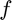with respect to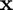at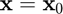in the direction of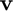using the forward difference approximation with a default relative step size of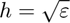, where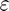is the machine zero.

Dv = fdirectional(f,x0,v,h) numerically evaluates the directional derivative ofwith respect toatin the direction ofusing the forward difference approximation with a user-specified relative step size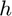.

## Input/Output Parameters

 Variable Symbol Description Format Input f multivariate, scalar-valued function () 1×1function_handle x0 evaluation point n×1double v vector defining direction of differentiation n×1double h (OPTIONAL) relative step size 1×1double Output Dv directional derivative of with respect to in the direction of , evaluated at 1×1double

## Note

• This function requires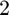evaluations of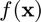.
• This implementation does not assume thatis a unit vector.

## Example

Approximate the directional derivative of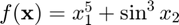at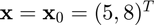in the direction of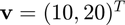using the fdirectional function, and compare the result to the true result of.

Approximating the directional derivative,

f = @(x) x(1)^5+sin(x(2))^3;
x0 = [5;8];
v = [10;20];
Dv = fdirectional(f,x0,v)

Dv =

3.1241e+04



Calculating the error,

error = Dv-(31250+60*sin(8)^2*cos(8))

error =

0.0019